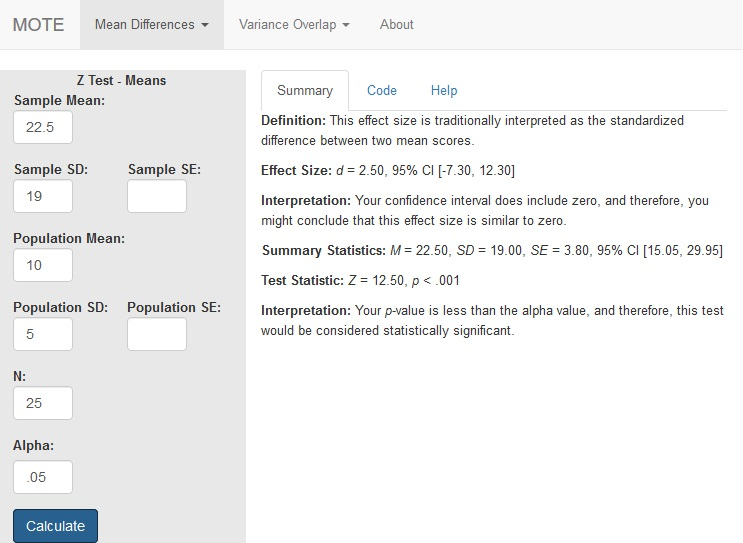# Description

This function displays $d_{z}$ for z-test with the population mean and standard deviation. The normal confidence interval is also provided.

The formula for $d_{z}$ is: $$d_{z} = \frac{M - \mu}{\sigma}$$

The formula for Z is: $$Z = \frac{M - \mu}{\sigma_M}$$

# R Function

d.z.mean(mu, m1, sig, sd1, n, a = 0.05)

# Arguments

• mu = population mean
• m1 = sample study mean
• sig = population standard deviation
• sd1 = standard deviation from the study
• n = sample size
• a = significance level

# Example

The average quiz test taking time for a 10 item test is 22.5 minutes, with a standard deviation of 10 minutes. My class of 25 students took 19 minutes on the test with a standard deviation of 5.

# Function in R:

d.z.mean(mu = 22.5, m1 = 19, sig = 10, sd1 = 5, n = 25, a = .05)

# MOTE

## Screenshot## Effect Size:

$d_{z}$ = 2.50, 95% CI [-7.30, 12.30]

## Interpretation:

Your confidence interval does include zero, and therefore, you might conclude that this effect size is similar to zero.

## Summary Statistics:

M = 22.50, SD = 19.00, SE = 3.80, 95% CI [15.05, 29.95]

## Test Statistic:

Z = 12.50, p < .001

## Interpretation:

Your p-value is less than the alpha value, and therefore, this test would be considered statistically significant.